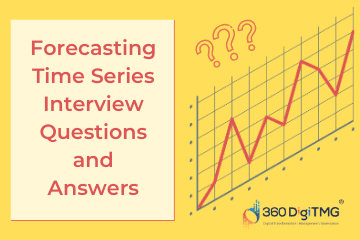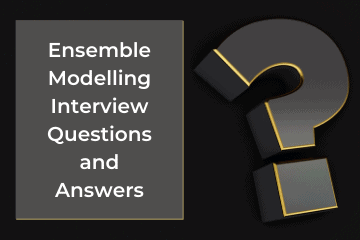Home / Blog / Interview Questions on Data Science / Lasso & Ridge Regression Interview Questions & Answers in 2023

# Lasso & Ridge Regression Interview Questions & Answers in 2023

• February 03, 2023
• 26788
• 39### Meet the Author : Mr. Bharani Kumar

Bharani Kumar Depuru is a well known IT personality from Hyderabad. He is the Founder and Director of Innodatatics Pvt Ltd and 360DigiTMG. Bharani Kumar is an IIT and ISB alumni with more than 17 years of experience, he held prominent positions in the IT elites like HSBC, ITC Infotech, Infosys, and Deloitte. He is a prevalent IT consultant specializing in Industrial Revolution 4.0 implementation, Data Analytics practice setup, Artificial Intelligence, Big Data Analytics, Industrial IoT, Business Intelligence and Business Management. Bharani Kumar is also the chief trainer at 360DigiTMG with more than Ten years of experience and has been making the IT transition journey easy for his students. 360DigiTMG is at the forefront of delivering quality education, thereby bridging the gap between academia and industry.

• ### Which of the following is a disadvantage of non-parametric machine learning algorithms?

• a) Capable of fitting a large number of functional forms (flexibility)
• b) Very fast to learn (speed)
• c) More of a risk to overfit the training data (overfitting)
• d) They do not require much training data

Answer - c) More of a risk to overfit the training data (overfitting)

• ### Which of the following is a true statement for regression methods the in case of feature selection?

• a) Ridge regression uses subset selection of features
• b) Lasso regression uses subset selection of features
• c) Both use subset selection of features
• d) None of above

Answer - b) Lasso regression uses subset selection of features

• a) True
• b) False

• a) 2
• b) 3
• c) 6
• d) Error

• a) True
• b) False

• ### Which regularization is used to reduce the over fit problem?

• a) L1
• b) L2
• c) Both
• d) None of the above

• ### To check the linear relationship of dependent and independent continuous variables, which of the following plots are best suited?

• a) Scatter plot
• b) Bar chart
• c) Histograms
• d) All of the above

• ### Statement 1: The cost function is altered by adding a penalty equivalent to the square of the magnitude of the coefficients Statement 2: Ridge and Lasso regression are some of the simple techniques to reduce model complexity and prevent overfitting which may result from simple linear regression.

• a) Statement 1 is true and statement 2 is false
• b) Statement 1 is False and statement 2 is true
• c) Both Statement (1 & 2) is true
• d) Both Statement (1 & 2) is wrong

Answer - c) Both Statement (1 & 2) is true

• ### What are the assumptions of linear regression?

• a) Output and input should be linear, dependent and parameters should be linear
• b) Multiple input variables should be non- linear, if multiple i/p variables linear that would be called co-linearity
• c) Error should be no-relation (or) error should be independent, if any relation b/w errors that is called Auto-Correlation
• d) All the above

Answer - d) All the above

• ### Which of the following of the coefficients is added as the penalty term to the loss function in Lasso regression?

• a) Squared magnitude
• b) Absolute value of magnitude
• c) Number of non-zero entries
• d) None of the above

Answer - b) Absolute value of magnitude

• ### What type of penalty is used on regression weights in Ridge regression?

• a) L0
• b) L1
• c) L2
• d) None of the above

• ### If two variables, x and y, have a very strong linear relationship, then

• a) There is evidence that x causes a change in y
• b) There is evidence that y causes a change in x
• c) There might not be any causal relationship between x and y
• d) None of these alternatives is correct

Answer - c) There might not be any causal relationship between x and y

• ### Which of the following is used for evaluating regression models?

• a) Adjusted R Squared, R Squared
• b) RMSE / MSE / MAE
• c) 1 is true and 2 is false
• d) Both 1 and 2 are true

Answer - d)Both 1 and 2 are true

• ### Lasso Regression uses which norm?

• a) L1.
• b) L2.
• c) L1 & L2 both.
• d) None of the above.

• ### Ridge Regression uses which norm?

• a) L1.
• b) L2.
• c) L1 & L2 both.
• d) None of the above.

• ### In Ridge regression, A hyper parameter is used called “_____________” that controls the weighting of the penalty to the loss function.

• a) Alpha.
• b) Gamma.
• c) Lambda.
• d) None of above.

• a) Lambda.
• b) Gamma.
• c) Beta.
• d) Alpha.

• ### Ridge regression can reduce the slope close to zero (but not exactly zero) but Lasso regression can reduce the slope to be exactly equal to zero.

• a) Both statements are True about Ridge and Lasso.
• b) Both statements are False about Ridge and Lasso.

• ### Ridge regression takes ________________ value of variables.

• a) Squared value of variables.
• b) Absolute value of variables.
• c) Cube value of variables.
• d) Root value of variables.

Answer - a) Squared value of variables

• ### Ridge regression takes ________________ value of variables.

• a) Squared value of variables.
• b) Absolute value of variables.
• c) Cube value of variables.
• d) Root value of variables.

Answer - b) Absolute value of variables

• ### )The effect of alpha value on both ridge and lasso regression is same in terms of value increase and decrease.

• a) True.
• b) False.
• c) alpha value is fix for ridge regression.
• d) None of the above.

• ### In this Lasso and Ridge regression as alpha value increases, the slope of the regression line reduces and becomes horizontal.

• a) Slope is fixed for whatever maybe the value.
• b) False.
• c) True.
• d) None of the above.

• ### The following statement is

I. Lasso Regression helps to reduce overfitting and it is particularly useful for feature selection.
II. Lasso regression can be useful if we have several independent variables that are useless.
• a) Statement ( I ) is true and statement ( II ) is false.
• b) Statement ( I ) is false and statement ( II ) is true.
• c) Both Statement ( I ) & ( II ) are wrong.
• D) Both Statement ( I ) & ( II ) are true.

Answer - D) Both Statement ( I ) & ( II ) are true

• a) Ggplot.
• b) Glmnet.
• c) Caret.
• d) Dplyr.

• ### With Lasso Regression the influence of the hyper parameter lambda, as lambda tends to zero the solution approaches to _________________ .

• a) Zero.
• b) One.
• c) Linear regression.
• d) Infinity.

• ### With Lasso Regression the influence of the hyper parameter lambda, as lambda tends to infinity the solution approaches to _________________ .

• a) Zero.
• b) One.
• c) Global mean.
• d) Infinity.

• ### When compared with Lasso regression, the Ridge regression works well in cases where we

• a) If we have more features.
• b) If we have less features.
• c) If features have high correlation.
• d) If features have low correlation.

Answer - b) If we have less features , c)If features have high correlation

• ### Suppose we fit “Lasso Regression” to a data set, which has 100 features (X1,X2…X100). Now, we rescale one of these feature by multiplying with 10 (say that feature is X1), and then refit Lasso regression with the same regularization parameter. Now, which of the following options will be correct?

• a) It is more likely for X1 to be excluded from the model
• b) It is more likely for X1 to be included in the model.
• c) Can’t say.
• d) None of these.

Answer - b) It is more likely for X1 to be included in the model

### Data Science Training Institutes in Other Locations